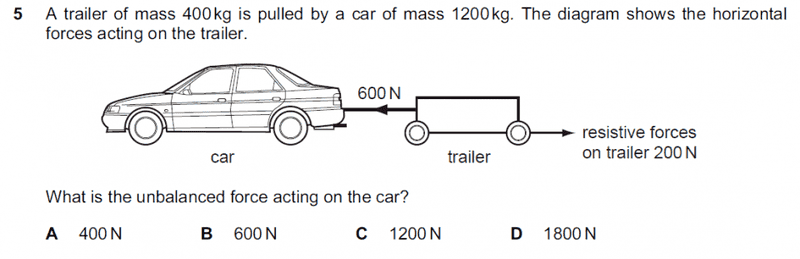# Unbalanced force acting on car

• toforfiltum

## Homework StatementF=ma

## The Attempt at a Solution

I figured that since car pulls on trailer with a force of 600 N and there is resistive force of 200 N, net force of car on trailer is 400 N. According to Newton's 3rd law, the unbalanced force acting on car would also be 400 N. But the answer is C. I have no clue how to get to that answer.

The 400kg trailer experiences a net forwards force of 400N. This translates into acceleration = 400 N / 400kg = 1 meter / second^2. The car accelerates at the same rate, so the net force on the car is f = 1200 kg x 1 meter / second^2 = 1200 N.

The driven tires on the car would have to exert 1200N + 600N = 1800 N backwards onto the ground, coexistent with the ground exerting 1800 N force forwards onto the driven tires and car.

•toforfiltum
The 400kg trailer experiences a net forwards force of 400N. This translates into acceleration = 400 N / 400kg = 1 meter / second^2. The car accelerates at the same rate, so the net force on the car is f = 1200 kg x 1 meter / second^2 = 1200 N.

The driven tires on the car would have to exert 1200N + 600N = 1800 N backwards onto the ground, coexistent with the ground exerting 1800 N force forwards onto the tires and car.
Ah, I see now. But may I know if the part I say above where trailer also exerts a force of 400 N is correct? If it isn't how is it correct without breaking Newton's 3rd law?

Newton third law pairs: Driven tires and pavement, 1800 N. Car and tow line, 600 N. Tow line and trailer, 600 N. The resisitve forces on the trailer would be due to internal friction of the bearing surfaces, rolling resistance, aerodynamic drag, ... .

I figured that since car pulls on trailer with a force of 600 N and there is resistive force of 200 N, net force of car on trailer is 400 N.

The net force on the trailer is 400 N, but the force on the trailer by the car is 600 N.

According to Newton's 3rd law, the unbalanced force acting on car would also be 400 N.

According to Newton's 3rd Law, the force on the car by the trailer is 600 N.

Newton third law pairs: Driven tires and pavement, 1800 N. Car and tow line, 600 N. Tow line and trailer, 600 N. The resisitve forces on the trailer would be due to internal friction of the bearing surfaces, rolling resistance, aerodynamic drag, ... .
Ok I see now. Thanks!
The net force on the trailer is 400 N, but the force on the trailer by the car is 600 N.

According to Newton's 3rd Law, the force on the car by the trailer is 600 N.
Thanks. I'm the one breaking the law.Hi toforfiltum, may I know what year is this paper from?Hi toforfiltum, may I know what year is this paper from?This not from A level. From Cambridge Pre-U.

This not from A level. From Cambridge Pre-U.
Oh ok thx